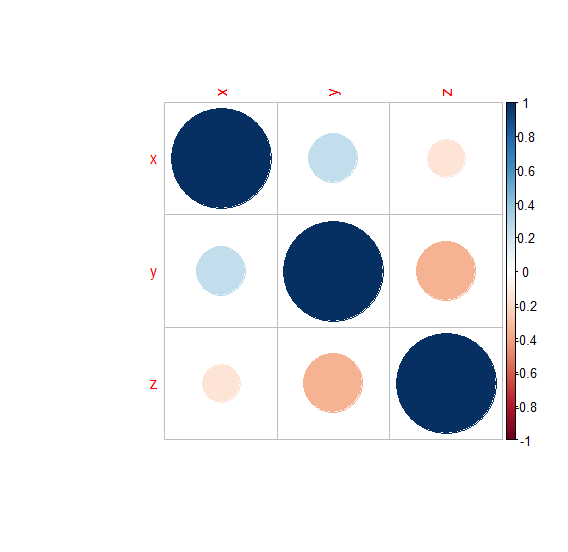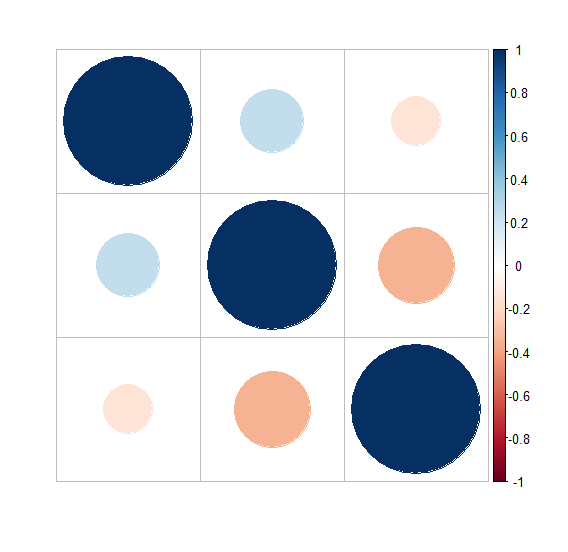# How to create correlation matrix plot without variables labels in R?

To create correlation matrix plot without variables labels in R, we can use tl.pos argument set to n.

For Example, if we have a correlation matrix say M then we can create the correlation matrix plot without variables labels by using the below command −

corrplot(M,tl.pos='n')

## Example

Following snippet creates a sample data frame −

x<-sample(0:9,20,replace=TRUE)
y<-sample(1:100,20)
z<-sample(101:1001,20)
df<-data.frame(x,y,z)
df

The following dataframe is created

   x  y   z
1 6 36 895
2 4 61 342
3 0 51 222
4 4 23 934
5 0 18 744
6 7 88 888
7 0 27 999
8 3 89 153
9 8 32 452
10 7 80 237
11 6 82 877
12 5 14 980
13 5 76 630
14 4 39 345
15 8 12 229
16 4 31 817
17 1 57 375
18 5 7  531
19 6 84 343
20 0  9 968

To find the correlation matrix for data in df on the above created data frame, add the following code to the above snippet −

x<-sample(0:9,20,replace=TRUE)
y<-sample(1:100,20)
z<-sample(101:1001,20)
df<-data.frame(x,y,z)
M<-cor(df)
M

## Output

If you execute all the above given snippets as a single program, it generates the following Output −

   x          y                 z
x  1.0000000  0.2435002 -0.1497751
y  0.2435002  1.0000000 -0.3495930
z -0.1497751 -0.3495930  1.0000000

To load corrplot package and creating correlation matrix plot on the above created data frame, add the following code to the above snippet −

x<-sample(0:9,20,replace=TRUE)
y<-sample(1:100,20)
z<-sample(101:1001,20)
df<-data.frame(x,y,z)
M<-cor(df)
library(corrplot)
corrplot(M)

## Output

If you execute all the above given snippets as a single program, it generates the following Output −To create correlation matrix plot without variables labels on the above created data frame, add the following code to the above snippet −

x<-sample(0:9,20,replace=TRUE)
y<-sample(1:100,20)
z<-sample(101:1001,20)
df<-data.frame(x,y,z)
M<-cor(df)
library(corrplot)
corrplot(M,tl.pos='n')

## Output

If you execute all the above given snippets as a single program, it generates the following Output −